NEET  >  DC Pandey Solutions: Gravitation - 1

# DC Pandey Solutions: Gravitation - 1 | Physics Class 11 - NEET

 1 Crore+ students have signed up on EduRev. Have you?

Introductory Exercise 10.1

Q.1. Calculate the change in the value of g at altitude 45°. Take radius of earth = 6.37 × 103 km
Sol.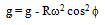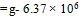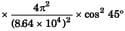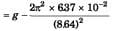= g -0.0168 m/s2
Dg = g-g' = 0.0168 m/s

Q.2. Determine the speed with which the earth would have to rotate on its axis, so that a person on the equator would weigh 3/5th as much as at present. Take R = 6400 km
Sol.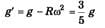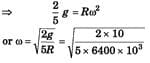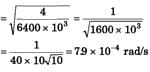Q.3. At what height from the surface of earth will the value of g be reduced by 36% from the value at the surface? R = 6400 km
Sol.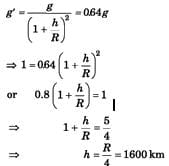Q.4. The distance between two bodies A and B is r. Taking the gravitational force according to the law of inverse square of r, the acceleration of the body A is a. If the gravitational force follows an inverse fourth power law, then what will be the acceleration of body A?
Sol.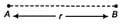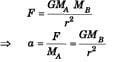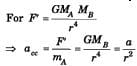Q.5. Find the force of attraction on a particle of mass m placed at the centre of a semicircular wire of length L and mass M.
Sol.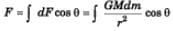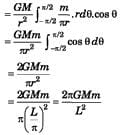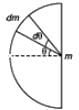Introductory Exercise 10.2

Q.1. A particle of mass m is placed at the centre of a uniform spherical shell of same mass and radius R. Find the gravitational potential at a distance R/2 from the centre.
Sol.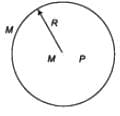V = V+ Vs =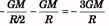Q.2. A particle of mass 20 g experiences a gravitational force of 4.0 N along positive x-direction. Find the gravitational field at that point.
Sol.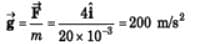200 N/kg i

Q.3. The gravitational potential due to a mass distribution is V = 3x2y + y3z. Find the gravitational field.
Sol.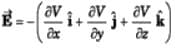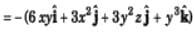Q.4. Gravitational potential at x = 2 m is decreasing at a rate of 10 J/kg-m along the positive x-direction. It implies that the magnitude of gravitational field at x = 2 m is also 10 N/kg. Is this statement true or false?

Sol. Only the variation is given along x-axis, nothing is about y and z axis, so, the statement is false.

Q.5. The gravitational potential in a region is given by, V = 20(x+ y) J/kg. Find the magnitude of the gravitational force on a particle of mass 0.5 kg placed at the origin.

Sol.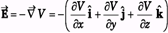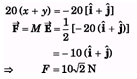Q.6. The gravitational field in a region is given by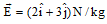Find the work done by the gravitational field when a particle of mass 1 kg is moved on the line 3y + 2x = 5 from (1 m, 1 m) to (-2 m, 3 m).

Sol.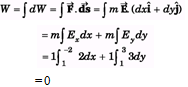The document DC Pandey Solutions: Gravitation - 1 | Physics Class 11 - NEET is a part of the NEET Course Physics Class 11.
All you need of NEET at this link: NEET

## Physics Class 11

127 videos|464 docs|210 tests

## Physics Class 11

127 videos|464 docs|210 tests

### How to Prepare for NEET

Read our guide to prepare for NEET which is created by Toppers & the best Teachers

Track your progress, build streaks, highlight & save important lessons and more!

,

,

,

,

,

,

,

,

,

,

,

,

,

,

,

,

,

,

,

,

,

;